0  447251  447259  447265  447269  447275  447277  447281  447287  447289  447295  447301  447305  447307  447311  447317  447319  447325  447329  447331  447335  447337  447341  447343  447345  447346  447347  447348

19.вҳ…(жң¬е°Ҹйўҳж»ЎеҲҶ10еҲҶ)е·ІзҹҘж•°еҲ—{an}гҖҒ{bn}йғҪжҳҜж— з©·зӯүе·®ж•°еҲ—,е…¶дёӯa1=3,b1=2,b2жҳҜa2дёҺa3зҡ„зӯүе·®дёӯйЎ№,дё”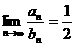.жұӮжһҒйҷҗ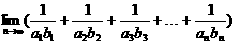зҡ„еҖј.

еҲҶжһҗ йҰ–е…ҲйңҖжұӮеҮәanгҖҒbnзҡ„иЎЁиҫҫејҸ,д»ҘзЎ®е®ҡжүҖжұӮжһҒйҷҗзҡ„иЎЁиҫҫејҸ,дёәжӯӨ,е…ій”®еңЁдәҺжұӮеҮәдёӨдёӘж•°еҲ—зҡ„е…¬е·®,вҖңb2жҳҜa2дёҺa3зҡ„зӯүе·®дёӯйЎ№вҖқе·Із»ҷеҮәдёҖдёӘзӯүйҮҸе…ізі»,вҖңanдёҺbnд№ӢжҜ”зҡ„жһҒйҷҗдёә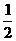вҖқеҸҲз»ҷеҮәдәҶеҸҰдёҖдёӘзӯүйҮҸе…ізі»,ж•…еҸҜиҖғиҷ‘е…Ҳи®ҫеҮәе…¬е·®з”ЁдәҢе…ғж–№зЁӢз»„жұӮи§Ј.

и§Ј и®ҫ{an}гҖҒ{bn}зҡ„е…¬е·®еҲҶеҲ«дёәd1гҖҒd2,

вҲө2b2=a2+a3,еҚі2(2+d2)=(3+d1)+(3+2d1),

вҲҙ2d2-3d1=2.в‘ гҖҖгҖҖ 2еҲҶ

еҸҲ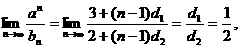еҚіd2=2d1,в‘ЎгҖҖгҖҖгҖҖ 4еҲҶ

иҒ”з«Ӣв‘ в‘Ўи§Јеҫ—d1=2,d2=4.

вҲҙan=a1+(n-1)d1=3+(n-1)В·2=2n+1,

bn=b1+(n-1)d2=2+(n-1)В·4=4n-2.гҖҖгҖҖ 6еҲҶ10еҲҶ

18.(жң¬е°Ҹйўҳж»ЎеҲҶ10еҲҶ)е·ІзҹҘж•°еҲ—{an}гҖҒ{bn},е…¶дёӯan=1+3+5+вҖҰ+(2n+1),bn=2n+4(nвүҘ5),иҜ•й—®жҳҜеҗҰеӯҳеңЁиҝҷж ·зҡ„иҮӘз„¶ж•°n,дҪҝеҫ—anвүӨbnжҲҗз«Ӣпјҹ

еҲҶжһҗ еҜ№nиөӢеҖјеҗҺ,жҜ”иҫғеҮ еҜ№anдёҺbnзҡ„еӨ§е°Ҹ,еҸҜдҪңеҮәеҗҲзҗҶзҢңжөӢ,еҶҚз”Ёж•°еӯҰеҪ’зәіжі•дәҲд»ҘиҜҒжҳҺ.

и§Ј an=1+3+5+вҖҰ+(2n+1)=(n+1)2,

еҪ“n=5ж—¶,a5=36,b5=25+4=36,жӯӨж—¶a5=b5;

еҪ“n=6ж—¶, a6=49,b6=26+4=68,жӯӨж—¶a6<b6;

еҪ“n=7ж—¶,a7=64,b7=27+4=132,жӯӨж—¶a7<b7;

еҪ“n=8ж—¶,a8=81,b8=28+4=260,жӯӨж—¶a8<b8.

зҢңжғі:еҪ“nвүҘ6ж—¶,жңүan<bn.гҖҖгҖҖгҖҖгҖҖ 3еҲҶ

дёӢйқўз”Ёж•°еӯҰеҪ’зәіжі•иҜҒжҳҺдёҠиҝ°зҢңжғі.

в‘ еҪ“n=6ж—¶,жҳҫз„¶дёҚзӯүејҸжҲҗз«Ӣ,вҲҙn=6ж—¶,дёҚзӯүејҸan<bnжҲҗз«Ӣ;

в‘ЎеҒҮи®ҫеҪ“n=k(kвүҘ6)ж—¶,дёҚзӯүејҸжҲҗз«Ӣ,еҚіak<bk,д№ҹеҚі(k+1)2<2k+4;еҪ“n=k+1ж—¶пјҢbk+1=2k+1+4=2(2k+4)-4>2(k+1)2-4=2k2+4k-2,

иҖҢ(2k2+4k-2)-(k+2)2=k2-6>0(вҲөkвүҘ6,вҲҙk2пјһ6),

еҚі2k2+4k-2>(k+2)2=пј»(k+1)+1пјҪ2.

з”ұдёҚзӯүејҸзҡ„дј йҖ’жҖ§,зҹҘbk+1>пј»(k+1)+1пјҪ2=ak+1.

вҲҙеҪ“n=k+1ж—¶,дёҚзӯүејҸд№ҹжҲҗз«Ӣ.гҖҖгҖҖ 8еҲҶ

з”ұв‘ в‘ЎеҸҜзҹҘ,еҜ№дёҖеҲҮnвҲҲN,дё”nвүҘ6,йғҪжңүan<bn.

з»јдёҠжүҖиҝ°,еҸҜзҹҘеҸӘжңүеҪ“n=5ж—¶,an=bn;еҪ“nвүҘ6ж—¶,anпјңbn.еӣ жӯӨеӯҳеңЁдҪҝanвүӨbnжҲҗз«Ӣзҡ„иҮӘз„¶ж•°n.

10еҲҶ

еҲҶжһҗ жң¬йўҳиҖғжҹҘз”Ёж•°еҲ—зҡ„йҖ’жҺЁе…¬ејҸжұӮйҖҡйЎ№еҸҠж•°еҲ—зҡ„жһҒйҷҗ.

и§Ј и®ҫз¬¬nж¬ЎеҺ»еҒҘиә«жҲҝзҡ„дәәж•°дёәan,еҺ»еЁұд№җе®Өзҡ„дәәж•°дёәbn,еҲҷan+bn=150,гҖҖгҖҖгҖҖгҖҖгҖҖгҖҖгҖҖ 2еҲҶ

вҲҙan=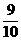an-1+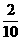bn-1=an-1+(150-an-1)=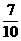an-1+30,

еҚіan=an-1+30.гҖҖгҖҖгҖҖгҖҖгҖҖгҖҖгҖҖгҖҖгҖҖ 4еҲҶ

вҲҙan-100=(an-1-100).дәҺжҳҜan-100=(a1-100)В·()n-1,еҚіan=100+()n-1В·(a1-100).гҖҖ 6еҲҶ

вҲҙ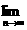an=100.ж•…йҡҸзқҖж—¶й—ҙзҡ„жҺЁз§»,еҺ»еҒҘиә«жҲҝзҡ„дәәж•°зЁіе®ҡеңЁ100дәәе·ҰеҸі.гҖҖгҖҖгҖҖгҖҖгҖҖгҖҖгҖҖ 8еҲҶ

жү«з ҒдёӢиҪҪдҪңдёҡзІҫзҒөеҗҢжӯҘз»ғд№ еҶҢзӯ”жЎҲ